# A guided tour to the MECS

## and to its response matrix

This set of HTML pages has been written by Lucio Chiappetti ( IFCTR)
with substantial input from the entire MECS team,
and in particular by Teresa Mineo and Cettina Maccarone (IFCAI).

# *. Table of Content

Navigation through the tour is possible in an ordered way using the Table of Content, or the activated navigation "buttons" at the end of each page, or randomly just following the links or clicking on images.

# 1. Introduction

We give here a quick description of the Medium Energy Concentrator Spectrometer (MECS)  on board the BeppoSAX satellite. In particular we give some information on the content of the various calibration files, their meaning and role (with particular, but not exclusive, regard to those entering the generation of the response matrix).

All values (even those inlined in the text, or plotted) will be produced on-line from the latest calibration files, in order to guarantee an automatic update.
Note that, for plots produced by IDL, we cannot guarantee availability of a free license at all times. If a plot cannot be produced, please try later.

## 1.1 MECS interactive map

The MECS consists of three units (hereafter named M1, M2, and M3), each composed of a grazing incidence Mirror Unit (MU), and of a position sensitive Proportional Counter (GSPC) located at the focal plane. The MUs are connected to the GSPCs by a carbon fiber envelope, ~2 m long.

The following interactive map gives an impression of the components of one MECS unit. You may click on the various items and labels to access information about the MECS components or the MECS calibration parameters.
Note that the vertical scale is magnified by about a factor 8 and some items have been exploded from their actual position for clarity. The carbon fiber optical envelope connecting mirrors and detector is not shown.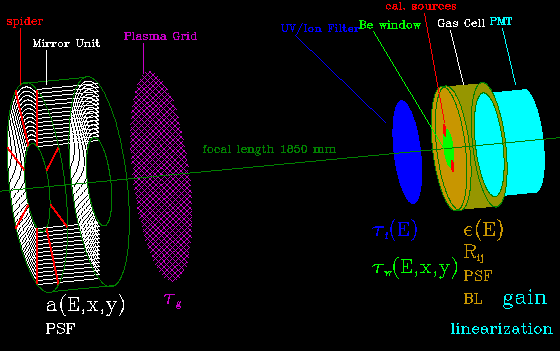Fig. 1.1-I : MECS interactive map

## 1.2 The response matrix : introduction

The response matrix of an X-ray detector allows to reconstruct source photon spectra from the observed counts (counts per Pulse Height Analyser [PHA] or Pulse Invariant [PI] channels). The detected count spectrum is in fact given by the convolution of the actual photon spectrum by the response matrix: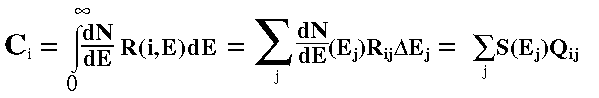(1)

[Click on each formula element to see its dimensions (physical units)]

where Ci are the detected counts in the i-th PI channel dN/dE is the photon input spectrum, R is the overall response matrix and j runs on a discrete binning of the photon input energy E, each j-th bin with DeltaEj width.
In formula (1) the matrix Qij corresponds to the XAS-format matrix file and has dimensions of [cm2 keV] however it is customary to use Rij as overall response matrix and to decompose it in two terms :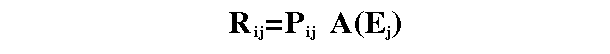(2)

where:

• Pij is the redistribution matrix (the adimensional probability that a photon of input energy Ej is detected in channel i) which correspond to an OGIP RMF file
• the 1-d array A(Ej) is the total effective area has dimensions of an area [cm2] and includes all contributions which depend only on the input photon energy and corresponds to an OGIP ARF file. For the MECS this is the overall effective area.
• (in the case of the MECS there are no terms which depend on the PHA/PI channel only)

### 1.2.1 Calibration data contributing to the redistribution matrix

The following (conventional or real) components enter in the computation of the response matrix :
The overall redistribution matrix can therefore be obtained as a sum of several terms (click on each formula component for details) as indicated below. Index k runs on the four main Xenon escape peaks.
Note that not all terms are defined in all energy ranges.
Also probabilities G and T are appropriately normalized for escape fractions in such a way that the total probability of a photon of energy Ej to spread over all channels is unity (except close to the extrema of the energy range where a photon may be lost out of the useful range).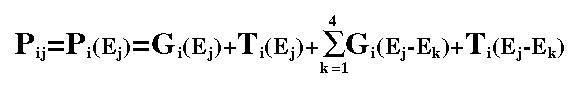(3)

A flow-chart of the calculation if the RMF in the XAS MECS matrix accumulation program is given in Fig. 1.2.1-I

### 1.2.2 Calibration data contributing to the overall effective area

The effective area as a function of energy is the product of several array or scalar terms. Some of these terms also depend on other parameters, like the position on the detector ,or the burst length limits.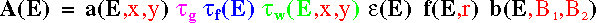(4)

The terms entering the above formula (click on each factor to access relevant information) are :

A flow-chart of the calculation if the RMF in the XAS MECS matrix accumulation program is given in Fig. 1.2.2-I

## 1.3 Calibration data used in event linearization

The position and energy of a photon are computed by the Electronics Unit based on the output of the PMT. This process introduces distortions both in position and gain because of three effects :
• spatial disuniformity of the PMT gain (energy independent)
• different viewing angles of different scintillation positions (energy independent)
• curvature of the Be window with associated distortion of the electric field (energy dependent)
The relevant distortions have been modelled using ground measurements based on a multipinhole mask ; the modeling is given as a relation converting unlinearized x,y pixel positions into linearized X,Y positions in mm, and as a map to correct for the position dependency of the gain.
Moreover, variations in the detector temperature produce sligthly changes in the gain that are corrected with respect to an absolute reference.

There are a number of calibration parameters that are typically used in corrections performed during data accumulation. We give here a quick reference list, with pointers to details kept elsewhere :

The final effect of the gain corrections is to convert PHA channels into PI channels.

## 1.4 Other calibration data

There are a number of calibration parameters which do not enter in the computation of the response matrix or in the corrections performed during data accumulation, but useful for the attitude reconstruction and data analysis. We give here a quick reference list, with pointers to details kept elsewhere :

## 1.5 MECS background

An invited paper  discussing the current knowledge of the analysis about the instrumental and cosmic background observed by the MECS has been presented at the RXTE/BeppoSAX Lincei conference "The Active X-ray sky" in Rome, 21-24 Oct 1997 (see preprint in astro-ph/9712251 or locally a preview)

The reader is referred to such paper for details. There are at present no standard XAS calibration files related to the background, although some material has been released by SDC. We recall here only the most important issues.

The background observed by the MECS is constituted by several components :

For the above reasons, it may not be wise to use as background subtraction the naive method of extracting gross source counts from a small circular region, and subtracting the background from a surrounding annulus. In fact the spectrum of the annulus is likely to be affected by the following effects :
• a non negligible fraction of the PSF of the source (a few percent) may be contaminating it
• the cosmic contribution will be underestimated because of vignetting effects
• the residual calibration events may be higher than in the detector centre
For these reasons it is advisable to subtract a blank field background accumulated in the same region where the source is extracted.